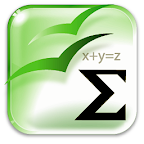Mathematical Formulae, Education

Mathematical FormulaeCategory: Education
Content Rating: Everyone
Instalations: 10,000 - 50,000
Version: 1.4
Requirements: Android 1.6 and up
Size: 13M
Update: 2013-04-30
Developer: YnotVcan
Description: The universe is fully dependent on mathematics. Here in "Mathematical Formulae" you can learn the basic formulas in mathematics. It is an offline app which acts as a portable library of significant mathematical formulas. The app covers the following:-? ALGEBRA? CALCULUS? GEOMETRY? TRIGONOMETRYThe further sub-section contains basic properties and methods to solve. You don't need to carry a formula book in your hand. Our commitments are respecting user privacy and delivering cool experience. Please help us by rating this app and recommending to the others. Tags : Ramanujam, Maths, Maths Formula, Mathematics, android Maths, Maths library, Maths Reference, Formulae, Analytical, Calculation, Problem Solving, mathematica, Math, Number

Rating: Tags: book geometry math